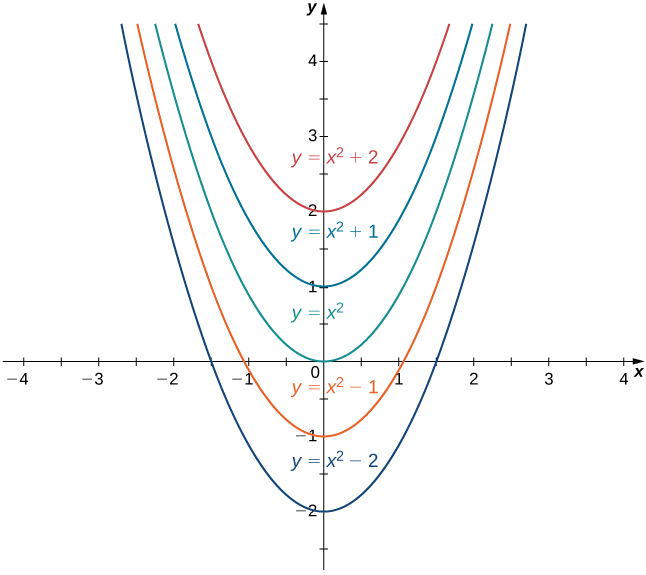# 4.10 Antiderivatives  (Page 2/10)

 Page 2 / 10

We use this fact and our knowledge of derivatives to find all the antiderivatives for several functions.

## Finding antiderivatives

For each of the following functions, find all antiderivatives.

1. $f\left(x\right)=3{x}^{2}$
2. $f\left(x\right)=\frac{1}{x}$
3. $f\left(x\right)=\text{cos}\phantom{\rule{0.1em}{0ex}}x$
4. $f\left(x\right)={e}^{x}$
1. Because
$\frac{d}{dx}\left({x}^{3}\right)=3{x}^{2}$

then $F\left(x\right)={x}^{3}$ is an antiderivative of $3{x}^{2}.$ Therefore, every antiderivative of $3{x}^{2}$ is of the form ${x}^{3}+C$ for some constant $C,$ and every function of the form ${x}^{3}+C$ is an antiderivative of $3{x}^{2}.$
2. Let $f\left(x\right)=\text{ln}|x|.$ For $x>0,f\left(x\right)=\text{ln}\left(x\right)$ and
$\frac{d}{dx}\left(\text{ln}\phantom{\rule{0.1em}{0ex}}x\right)=\frac{1}{x}.$

For $x<0,f\left(x\right)=\text{ln}\left(\text{−}x\right)$ and
$\frac{d}{dx}\left(\text{ln}\left(\text{−}x\right)\right)=-\frac{1}{\text{−}x}=\frac{1}{x}.$

Therefore,
$\frac{d}{dx}\left(\text{ln}|x|\right)=\frac{1}{x}.$

Thus, $F\left(x\right)=\text{ln}|x|$ is an antiderivative of $\frac{1}{x}.$ Therefore, every antiderivative of $\frac{1}{x}$ is of the form $\text{ln}|x|+C$ for some constant $C$ and every function of the form $\text{ln}|x|+C$ is an antiderivative of $\frac{1}{x}.$
3. We have
$\frac{d}{dx}\left(\text{sin}\phantom{\rule{0.1em}{0ex}}x\right)=\text{cos}\phantom{\rule{0.1em}{0ex}}x,$

so $F\left(x\right)=\text{sin}\phantom{\rule{0.1em}{0ex}}x$ is an antiderivative of $\text{cos}\phantom{\rule{0.1em}{0ex}}x.$ Therefore, every antiderivative of $\text{cos}\phantom{\rule{0.1em}{0ex}}x$ is of the form $\text{sin}\phantom{\rule{0.1em}{0ex}}x+C$ for some constant $C$ and every function of the form $\text{sin}\phantom{\rule{0.1em}{0ex}}x+C$ is an antiderivative of $\text{cos}\phantom{\rule{0.1em}{0ex}}x.$
4. Since
$\frac{d}{dx}\left({e}^{x}\right)={e}^{x},$

then $F\left(x\right)={e}^{x}$ is an antiderivative of ${e}^{x}.$ Therefore, every antiderivative of ${e}^{x}$ is of the form ${e}^{x}+C$ for some constant $C$ and every function of the form ${e}^{x}+C$ is an antiderivative of ${e}^{x}.$

Find all antiderivatives of $f\left(x\right)=\text{sin}\phantom{\rule{0.1em}{0ex}}x.$

$\text{−}\text{cos}\phantom{\rule{0.1em}{0ex}}x+C$

## Indefinite integrals

We now look at the formal notation used to represent antiderivatives and examine some of their properties. These properties allow us to find antiderivatives of more complicated functions. Given a function $f,$ we use the notation ${f}^{\prime }\left(x\right)$ or $\frac{df}{dx}$ to denote the derivative of $f.$ Here we introduce notation for antiderivatives. If $F$ is an antiderivative of $f,$ we say that $F\left(x\right)+C$ is the most general antiderivative of $f$ and write

$\int f\left(x\right)dx=F\left(x\right)+C.$

The symbol $\int$ is called an integral sign , and $\int f\left(x\right)dx$ is called the indefinite integral of $f.$

## Definition

Given a function $f,$ the indefinite integral    of $f,$ denoted

$\int f\left(x\right)dx,$

is the most general antiderivative of $f.$ If $F$ is an antiderivative of $f,$ then

$\int f\left(x\right)dx=F\left(x\right)+C.$

The expression $f\left(x\right)$ is called the integrand and the variable $x$ is the variable of integration .

Given the terminology introduced in this definition, the act of finding the antiderivatives of a function $f$ is usually referred to as integrating $f.$

For a function $f$ and an antiderivative $F,$ the functions $F\left(x\right)+C,$ where $C$ is any real number, is often referred to as the family of antiderivatives of $f.$ For example, since ${x}^{2}$ is an antiderivative of $2x$ and any antiderivative of $2x$ is of the form ${x}^{2}+C,$ we write

$\int 2x\phantom{\rule{0.1em}{0ex}}dx={x}^{2}+C.$

The collection of all functions of the form ${x}^{2}+C,$ where $C$ is any real number, is known as the family of antiderivatives of $2x.$ [link] shows a graph of this family of antiderivatives.The family of antiderivatives of 2 x consists of all functions of the form x 2 + C , where C is any real number.

For some functions, evaluating indefinite integrals follows directly from properties of derivatives. For example, for $n\ne \text{−}1,$

$\int {x}^{n}dx=\frac{{x}^{n+1}}{n+1}+C,$

which comes directly from

$\frac{d}{dx}\left(\frac{{x}^{n+1}}{n+1}\right)=\left(n+1\right)\frac{{x}^{n}}{n+1}={x}^{n}.$

This fact is known as the power rule for integrals .

## Power rule for integrals

For $n\ne \text{−}1,$

$\int {x}^{n}dx=\frac{{x}^{n+1}}{n+1}+C.$

Evaluating indefinite integrals for some other functions is also a straightforward calculation. The following table lists the indefinite integrals for several common functions. A more complete list appears in Appendix B .

#### Questions & Answers

Find the derivative of g(x)=−3.
Abdullah Reply
any genius online ? I need help!!
Guzorochi Reply
how can i help you?
Pina
need to learn polynomial
Zakariya
i will teach...
nandu
I'm waiting
Zakariya
plz help me in question
Abish
evaluate the following computation (x³-8/x-2)
Murtala Reply
teach me how to solve the first law of calculus.
Uncle Reply
what is differentiation
Ibrahim Reply
f(x) = x-2 g(x) = 3x + 5 fog(x)? f(x)/g(x)
Naufal Reply
fog(x)= f(g(x)) = x-2 = 3x+5-2 = 3x+3 f(x)/g(x)= x-2/3x+5
diron
pweding paturo nsa calculus?
jimmy
how to use fundamental theorem to solve exponential
JULIA Reply
find the bounded area of the parabola y^2=4x and y=16x
Omar Reply
what is absolute value means?
Geo Reply
Chicken nuggets
Hugh
🐔
MM
🐔🦃 nuggets
MM
(mathematics) For a complex number a+bi, the principal square root of the sum of the squares of its real and imaginary parts, √a2+b2 . Denoted by | |. The absolute value |x| of a real number x is √x2 , which is equal to x if x is non-negative, and −x if x is negative.
Ismael
find integration of loge x
Game Reply
find the volume of a solid about the y-axis, x=0, x=1, y=0, y=7+x^3
Godwin Reply
how does this work
Brad Reply
Can calculus give the answers as same as other methods give in basic classes while solving the numericals?
Cosmos Reply
log tan (x/4+x/2)
Rohan
please answer
Rohan
y=(x^2 + 3x).(eipix)
Claudia
is this a answer
Ismael
A Function F(X)=Sinx+cosx is odd or even?
WIZARD Reply
neither
David
Neither
Lovuyiso
f(x)=1/1+x^2 |=[-3,1]
Yuliana Reply
apa itu?
fauzi

### Read also:

#### Get Jobilize Job Search Mobile App in your pocket Now!

Source:  OpenStax, Calculus volume 1. OpenStax CNX. Feb 05, 2016 Download for free at http://cnx.org/content/col11964/1.2
Google Play and the Google Play logo are trademarks of Google Inc.

Notification Switch

Would you like to follow the 'Calculus volume 1' conversation and receive update notifications?By Christine ZeelieBy Royalle MooreBy OpenStaxBy Marion CabalfinBy Sam LuongBy Rebecca ButterfieldBy Frank LevyBy Mackenzie WilcoxBy John GabrieliBy Ryan Lowe